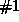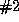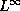Next: Introduction Up: Approximate Solutions of Nonlinear Previous: Contents

# Non-oscillatory central schemes

### Abstract:

We discuss a new class of high-resolution approximations for hyperbolic systems of conservation laws, which are based on central differencing. Its two main ingredients include:. A non-oscillatory reconstruction of pointvalues from their given cell averages; and. A central differencing based on staggered evolution of the reconstructed averages.

Many of the modern high-resolution schemes for such systems, are based on Godunov-type upwind differencing; their intricate and time consuming part involves the field-by-field characteristic decomposition, which is required in order to identify the "direction of the wind". Instead, our proposed central (staggered) stencils enjoy the main advantage is simplicity: no Riemann problems are solved, and hence field-by-field decompositions are avoided. This could be viewed as the high-order sequel to the celebrated Lax-Friedrichs (staggered) scheme. Typically, staggering suffers from excessive numerical dissipation. Here, excessive dissipation is compensated by using modern, high-resolution, non-oscillatory reconstructions.

We highlight several features of this new class of central schemes.

Scalar equations. For both the second- and third-order schemes we prove variation bounds (- which in turn yield convergence with precise error estimates), as well as entropy and multidimensional-stability estimates.

Systems of equations. Extension to systems is carried out by componentwise application of the scalar framework. It is in this context that our central schemes offer a remarkable advantage over the corresponding upwind framework.

Multidimensional problems. Since we bypass the need for (approximate) Riemann solvers, multidimensional problems are solved without dimensional splitting. In fact, the proposed class of central schemes is utilized for a variety of nonlinear transport equations.

A variety of numerical experiments confirm the high-resolution content of the proposed central schemes. They include second- and third-order approximations for one- and two-dimensional Euler, MHD, as well as other compressible and incompressible equations. These numerical experiments demonstrate that the proposed central schemes offer simple, robust, Riemann-solver-free approximations, while at the same time, they retain the high-resolution content of the more expensive upwind schemes.Next: Introduction Up: Approximate Solutions of Nonlinear Previous: Contents